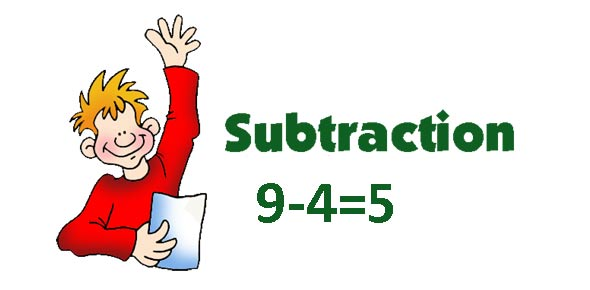# Subtraction

11 Questions | Total Attempts: 69SettingsThis is intended for Grade 1 puppils of DLSZ.

Related Topics
• 1.
In subtraction, regrouping means borrowing.
• A.

True

• B.

False

• 2.
Regrouping is done whenever a column has a smaller number on top as in this example. 33-153 is smaller than 5.
• A.

True

• B.

False

• 3.
Which shows renaming of 54?
• A.

50 + 14

• B.

40 + 14

• C.

50 + 4

• 4.
Which shows regrouping of 54
• A.

40 + 5

• B.

40 + 14

• C.

50 + 4

• 5.
We can change the order of the minuend and the subtrahend.
• A.

True

• B.

False

• 6.
Subtrahend is ...
• A.

The number we take away

• B.

The result of subtraction

• C.

The number from which another number is to be subtracted

• 7.
Minuend is
• A.

The number we take away

• B.

The number from which another number is to be subtracted

• C.

The result of subtraction

• 8.
In 50 -24 = 26, 50 is the ...
• A.

Minuend

• B.

Subtrahend

• C.

Difference

• 9.
The difference of 45 - 21 is ...
• A.

23

• B.

24

• C.

25

• 10.
30 + 16 is regroup d what number.
• A.

46

• B.

45

• C.

54

• 11.
Which is correct?
• A.

50 - 13 = 37

• B.

50 - 13 = 38

• C.

47 - 19 = 19